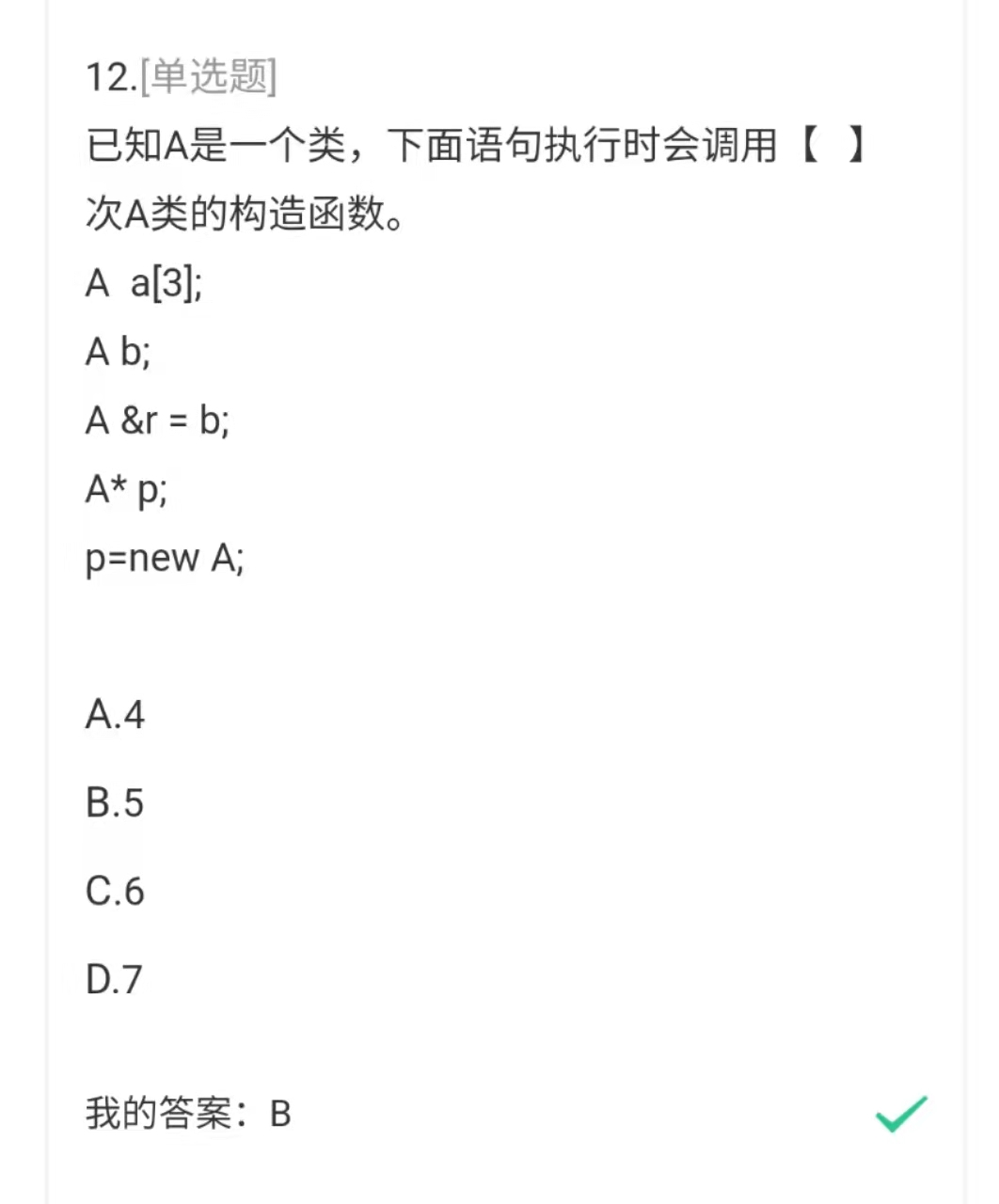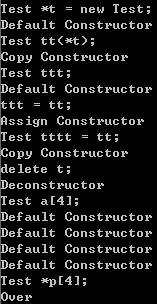2021-05-27 16:00

# 调用构造函数次数，new的用法？• 点赞
• 写回答
• 关注问题
• 收藏
• 邀请回答

#### 3条回答默认 最新

•小P聊技术 2021-05-27 16:03
已采纳

于构造函数的调用次数，这里有个小总结：
简单粗暴

测试代码：

``````#include <iostream>
using namespace std;
class Test{
int a;
public:
Test(){
a = 0;
cout << "Default Constructor" << endl;
}

Test(Test &t){
cout << "Copy Constructor" << endl;
a = t.a;
}

Test& Test :: operator = (const Test &t){
cout << "Assign Constructor" << endl;
if (&t == this)
return *this;
a = t.a;
return *this;
}
~Test(){
cout << "Deconstructor" << endl;
}
};

int main(){
cout << "Test *t = new Test;" << endl;
Test *t = new Test;
cout << "Test tt(*t);" << endl;
Test tt(*t);
cout << "Test ttt;" << endl;
Test ttt;
cout << "ttt = tt;" << endl;
ttt = tt;
cout << "Test tttt = tt;" << endl;
Test tttt = tt;
cout << "delete t;" << endl;
delete t;
cout << "Test a;" << endl;
Test a;
cout << "Test *p;" <<endl;
Test *p;
cout << "Over" << endl;
system("pause");
return 0;
}
``````

运行结果：注意：类声明指针的时候不调用构造函数。

补充一下思路：

1. Test *t = new Test;
调用默认构造函数：通过new创建一个对象，new的底层是会调用类的构造函数的，在堆上动态分配一段内存，并将指针t指向这段内存地址。由于这里没有参数传递，所以只调用的是默认构造函数。
2. Test tt(*t);
用括号中的内容来初始化Test类型的对象tt，调用拷贝构造函数。这里涉及到一个知识点，拷贝构造函数传入的参数必须是对象的引用，避免循环拷贝现象的发生。
3. Test ttt;
定义一个Test类型的对象ttt，并且没有为其赋初值，调用默认构造函数在栈区申请一段内存空间。
4. ttt = tt;
用tt对象给ttt对象赋值，这里的赋值运算符实际上是调用了赋值构造函数。注意：此处是先对ttt进行定义，为其申请了一段内存空间，这里是对ttt进行赋值操作。
5. Test tttt = tt;
此处与上一行不同的是，这里是同时进行变量tttt的定义和初始化操作，因此这里虽然有赋值运算符=在，但是其实质还是初始化，并不属于赋值的范畴，因此这里调用的是复制构造函数，而非赋值构造函数。
6. delete t;
without any problem，这里delete会调用对应的析构函数。
7. Test a;
这里定义了一个存放有4个Test类型对象的数组，因此会调用4次默认构造函数。
8. Test *p;
类声明指针的时候不调用构造函数。
点赞 1 打赏 评论
•秋杪 2021-05-27 16:06

A a 这里会调用3次；

A b 这里调用1次；

最后new调用一次；

单独新建类指针和引用是不会调构造函数的

点赞 打赏 评论
•qfl_sdu 2021-05-27 16:07

5次。a这3次，b一次，r是b的引用，没有构造，p在new的时候一次，所以一共5次。

点赞 打赏 评论